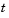MathematicsPDE Function Summary

MATLAB PDE Solver

This is the MATLAB PDE solver.

 PDE Initial-Boundary Value Problem Solver `pdepe` Solve initial-boundary value problems for systems of parabolic and elliptic PDEs in one space variable and time.

PDE Helper Function

 PDE Helper Function `pdeval` Evaluate the numerical solution of a PDE using the output of `pdepe`.

PDE Examples

These examples illustrate some problems you can solve using the MATLAB PDE solver. Click the example name to see the code in an editor. Type the example name at the command line to run it.

 Note    The Differential Equations Examples browser enables you to view the code for the PDE examples, and also run them. Click on the link to invoke the browser, or type `odeexamples('pde')`at the command line.

 Example Description `pdex1` Simple PDE that illustrates the straightforward formulation, computation, and plotting of the solution `pdex2` Problem that involves discontinuities `pdex3` Problem that requires computing values of the partial derivative `pdex4` System of two PDEs whose solution has boundary layers at both ends of the interval and changes rapidly for small`pdex5` System of PDEs with step functions as initial conditionsPartial Differential Equations Introduction to PDE Problems© 1994-2005 The MathWorks, Inc.